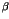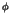Next: 4.5.3.14.1 Coefficients for Boundary Up: 4.5.3 PROMIS-NT Model Classes Previous: 4.5.3.13.2 Vector Coefficients for

### 4.5.3.14 Coefficients for Boundary Models

The coefficientsandin (4.7) - (4.8) are computed with Models derived from the classes CoeffModel_beta and CoeffModel_phi respectively. Quantity values determined by Dirichlet type boundary conditions (4.9) - (4.10) are computed by Models derived form the base class DirichletModel. The naming conventions of their Interface Parameters are similar to the according conventions for segment models described in Section 4.5.3.13. Different is that each quantity is represented by two values assigned to the two material segments forming the boundary. In this context the material segment indexed with '1' represents the segment in the first array index of the boundaryModel Parameter of the PromisNTSetupModel instance (Section 4.5.3.5).

Robert Mlekus
1999-11-14